In order to simplify radical expressions, you need to be aware of the following rules and properties of radicals 1) From definition of n th root(s) and principal root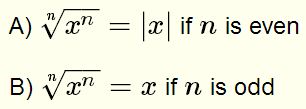ExamplesMore examples on Roots of Real Numbers and Radicals. 2) Product (Multiplication) formula of radicals with equal indices is given byMore examples on how to Multiply Radical Expressions. 3) Quotient (Division) formula of radicals with equal indices is given byMore examples on how to Divide Radical Expressions. 4) You may add or subtract like radicals only ExampleMore examples on how to Add Radical Expressions. 5) You may rewrite expressions without radicals (to rationalize denominators) as follows A) Example 1:B) Example 2: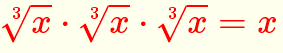C) Example 3:More examples on how to Rationalize Denominators of Radical Expressions. Examples Rationalize and simplify the given expressionsAnswers to the above examples 1) Write 128 and 32 as product/powers of prime factors: 128 = 27 , 32 = 25 hence2) Use product rule to write that √2 √6 = √123) Write 14 and 63 as products of prime numbers 14 = 2 � 7 , 63 = 32 � 7 and substitute4) Write 32 and 16 as products of prime numbers 32 = 2 5 , 16 = 2 4 and substitute5) Write 64 as products of prime numbers 64 = 2 6 and substituteRationalize the denominator by multiplying numerator and denominator by (3√7)26) Write 54 as products of prime numbers 54 = 2 � 3 3 and substitute7) Multiply the denominator and numerator by the conjugate of the denominatorExpand and simplifyMore Questions With Answers Use all the rules and properties of radicals to rationalize and simplify the following expressions.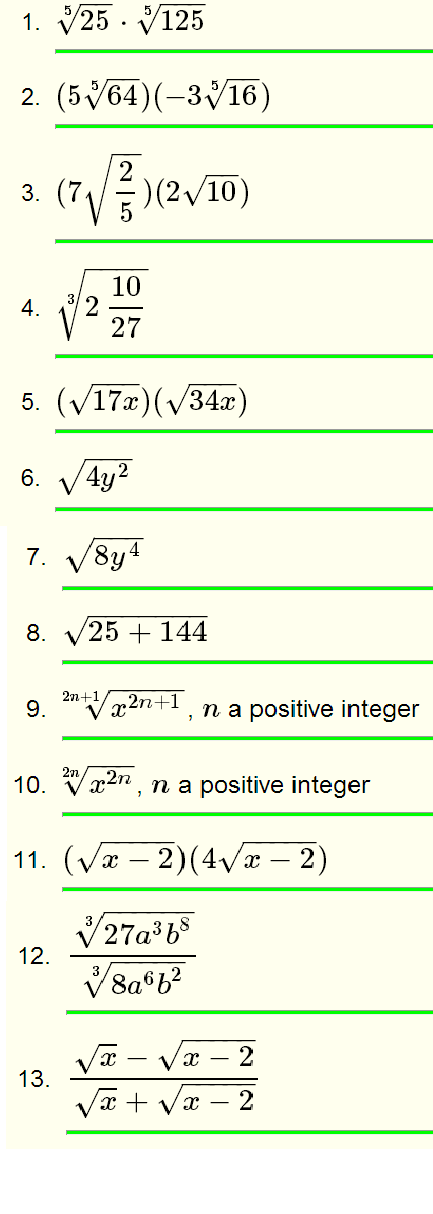Solutions to the Above Questions Write 25 and 125 as the product of prime factors: 25 = 52 and 125 = 53, henceWrite 64 and 16 as the product of prime factors: 64 = 26 and 16 = 24, henceUse product ruleConvert the mixed number under the radical into a fraction and substituteUse the division formula for radicalsWrite 64 and 27 as product of prime factors, substitute and simplifyUse the product formula and write 34 as the product of prime factors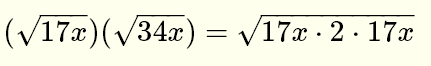SimplifyFor √(17 x) and √(34 x) to be real numbers, x must be positive hence |x| = xWrite the radicand as a square and simplify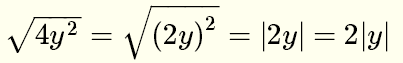Write the radicand as the product of $2$ and a square and simplifySimplify the radicand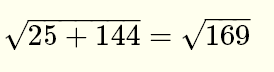Write as the product of prime factors and simplifySince n is a positive integer, then N = 2 n + 1 is an odd integer. HenceSince n is a positive integer, then N = 2 n is an even integer. Hence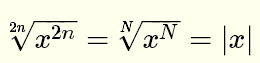Use division rule and simplify the radicandMultiply numerator and denominator by the conjugate of the denominatorExpand and simplify